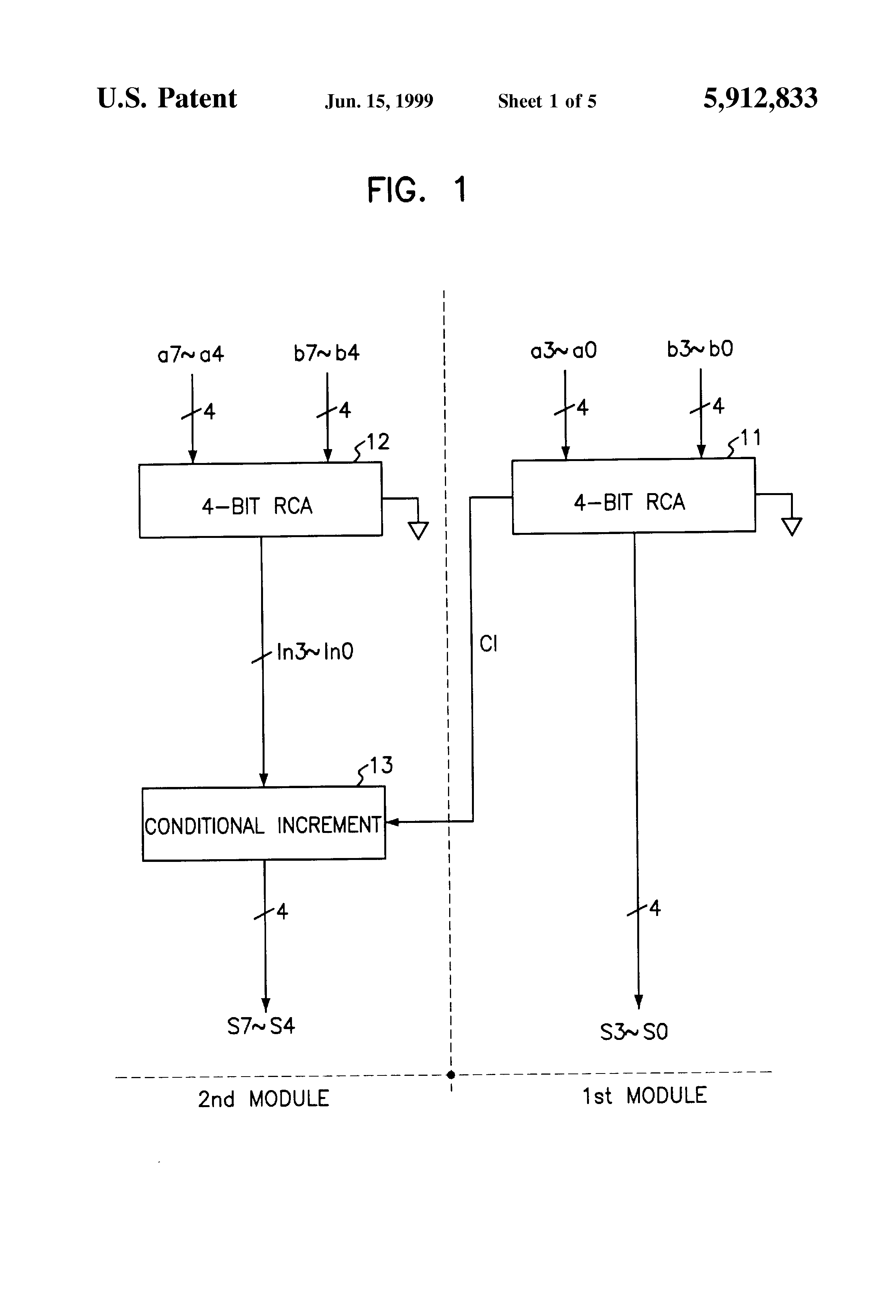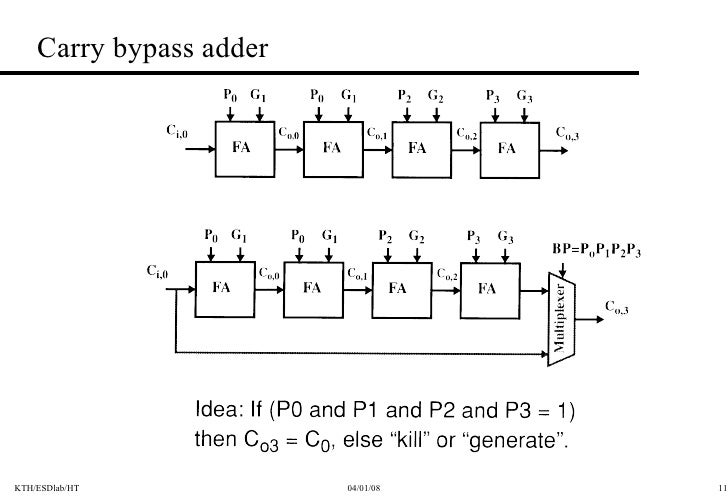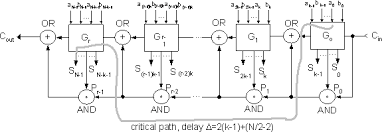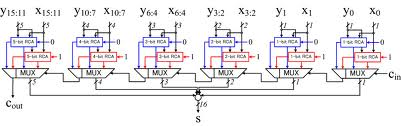﻿ DESIGN AND PERFORMANCE ANALYSIS OF VARIOUS ADDERSBack

## DESIGN AND PERFORMANCE ANALYSIS OF VARIOUS ADDERSArithmetic unit are the essential blocks of digital systems such as Digital Signal Processor (DSP),microprocessors, microcontrollers, and other data processing units. Adders become a critical hardware unit forthe efficient implementation of arithmetic unit. In many arithmetic applications and other kinds of applications,adders are not only in the arithmetic logic unit, but also in other parts of processor. Addition operation can alsobe used in complement operations (1’s, 2’s, and so on), encoding, decoding and so on. In general, addition is aprocess which involves two numbers which are added and carry will be generated. The addition operations willresult in sum value and carry value. All complex adder architectures are constructed from its basic buildingblocks such as Half Adder (HA) and Full Adder (FA).In this paper, an attempt has been made to design andsimulate the different types of adders using Verilog language and Xilinix ISE 13.2. Then the performanceparameters of the various adders are calculated and compared. The rest of the paper is organised as follows:Section I deals with the introduction about adders. In section II, the designs and the features of various addersare discussed. And section III deals with the simulation and synthesis results of adders.The designs of various adders are  as follows:

• Its an basic adder which  works on basic logic principle.
• The two exclusive-OR gate performe an sum of two single bit addition.
• And three  AND gate and one OR gate  perform the carry for two single bit.
• The combination of these function is known as FULL ADDER(FA)  unit.
• These FA unit can connect serially to achive number of addition.
• The carry can ripple through each FA unit to produce the final arithmetic results.
•  So the delay is more as the number of bits increases.
• Hence its known Riiple Carry Adder.
• AREA (LUT)=8;
• DELAY(ns) =2.191.• This adder is designed to speed up arithmetic operation by adding the propagation of carry bit.
• The carry skip circuitry consists of AND gate accept the carry-in bit and compare with group of  propagted signals.
• It uses skip logic in propagation of carry.
• The delay is high compared with RCA.
• This is one of  the efficient adder design
• Hence its known as Carry Skip Adder.
• AREA (LUT)=8;
• DELAY(ns) =2.296.• This adder is designed with the combination of Ripple Carry Adder and incrementation circuitry.
• The incrementation circuitry is design using Half Adder in Ripple Carry Chain in a sequential order.
• The Addition operation is done by dividing the total  number of bits into number of groups
• This is one of the  efficient adder design.
• Delay is less compared with RCA
• AREA (LUT)=8.
• DELAY(ns) =1.907.• This  adder is design with the Ripple Carry Adder  and By-pass circuitry.
• During number of bit addition carry generated will be propagated to next stage with help of multiplexer using select input as By-pass logic.
• Depending on the carry value and By-pass logic, the carry is propagated to the next FA unit.
• By-pass logic is formed from the product values as it is calculated in the CLA.
• Delay is high compared with RCA adder.
• AREA(LUT) =12.
• DELAY(ns)  =3.160.• This adder design is based on the principle of looking at lower adder bits of argument and addend if higher orders carry generated.
• This adder reduces the carry delay by reducing the number of gates through which a carry signal must propagate.
• The generation and propagation stage,the generation values, propagation values are computed.
• Internal carry generation is calculated in second stage. and in final stage, the sum is calculated.
• AREA(LUT) =10.
• DELAY(ns) =2.266.• The number of bits are added parallely at same time.
• The carry is not propagated through the stages and the carry is stored in present stage.
• Hence, the delay due to the carry is reduced.
• AREA (LUT)=13.
• DELAY(ns) =1.433.• The Architecture consists of independent generation of sum and carry i.e., Cin=1
and Cin=0 are executed parallelly
• Depending upon Cin, the external multiplexers select the carry to be propagated.
• Based on the carry input, the sum will be selected.
• Hence, the delay is reduced.
• However, the structure is increased due to the complexity of multiplexers.
• AREA(LUT) =8.
• DELAY(ns) =2.588.